# Measuring High-Frequency, Common-Mode Current, Voltage, and Impedance (Part II)Get valuable resources straight to your inbox - sent out once per month

## Introduction

This is the second part of a three-part series discussing measurement methods for high-frequency, common-mode (CM) current, voltage, and impedance using a flyback converter. Part I covered the basics of radiated EMI and the traditional method for measuring CM current in a flyback converter topology. Part II will propose a solution to common errors when measuring CM current, and then it will demonstrate how to measure CM impedance. In Part III, we will examine the switching source effect and equivalent voltage source.

## Magnetic Ring Solution for Measuring CM Current

Previously, we introduced the traditional method to measure the CM current, as well as its common pitfalls. In particular, there are two types of measurement errors. Figure 1 shows the error caused by electric field coupling, which is also known as the near-field coupling effect.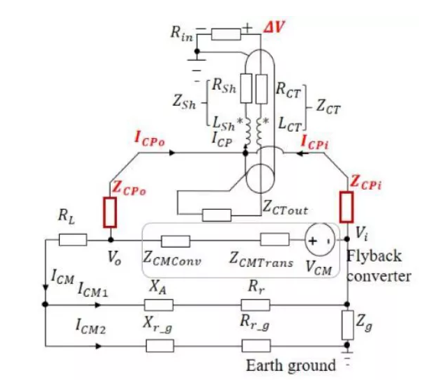Figure 1: Near-Field Coupling Effect in CM Current Testing

Figure 2 shows the error caused by the input line’s ground impedance (ZG). ZG is the impedance between the zero line and ground, and it changes depending on the environment. This impedance loop acts as a CM current shunt, leading to inconsistent test results in different environments.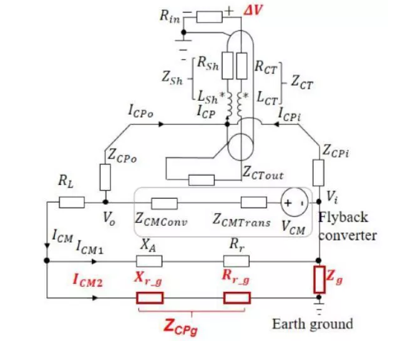Figure 2: Ground Impedance Effect in CM Current Testing

To solve these measurement errors, it is recommended to add multiple ferrite beads to the front of the coaxial line and the input line (see Figure 3).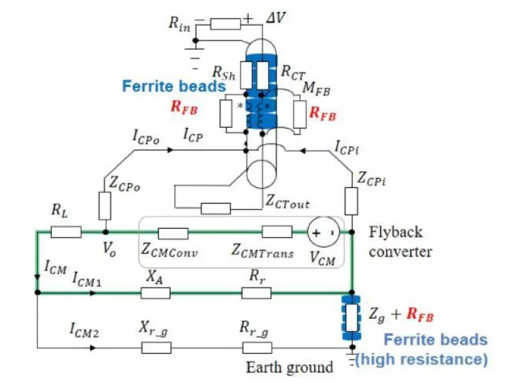Figure 3: Improved CM Current Test Method with Ferrite Beads

The ferrite bead can provide an impedance up to several thousand Ohms within a 30MHz to 1GHz radiation frequency range, effectively avoiding the influence of coupling and ground impedance.

Figure 4 shows a comparison of the CM current test results with or without a ferrite bead. If a ferrite bead is not added, then the measured CM current is significantly higher due to the near-field interference, which results in an error of up to tens of dB.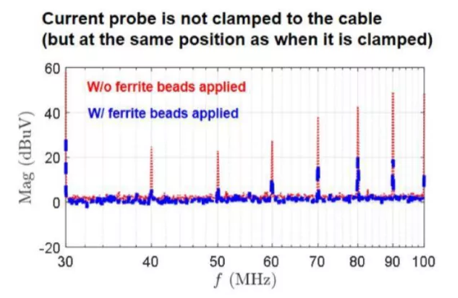Figure 4: Comparison of CM Current Test Results

Figure 5 shows measurement errors due to coupling. With the addition of ferrite beads, the measured CM current is more accurate.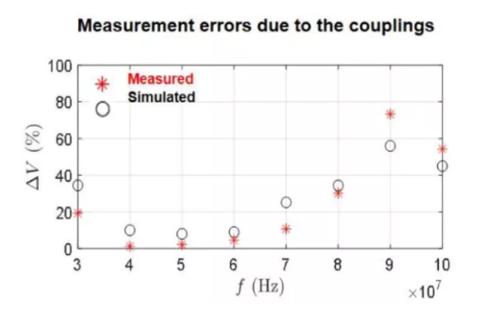Figure 5: Coupling Measurement Errors

## Measuring CM Impedance in Flyback Converters

The flyback converter’s CM impedance mainly refers to the transformer’s CM impedance (ZCMTRANS). This means is it necessary to know how to obtain the CM impedance.

Figure 6 shows how the switches in the flyback’s EMI model can be replaced with voltage sources. The voltages for the primary-side and secondary-side switches are VPRI and VSEC, respectively. Using the superposition theorem, we can evaluate the contribution of these two sources to the CM current. The radiated EMI model considers VPRI as a CM noise source. ZCMTRANS is the impedance in the Thevenin equivalent model between the primary and secondary sides, and VCM is the voltage source in the Thevenin equivalent model.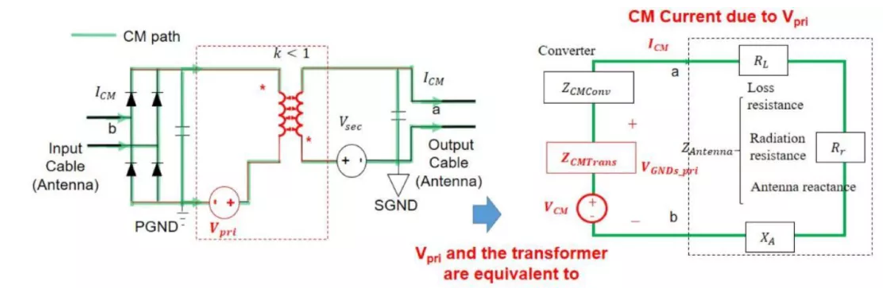Figure 6: Substitution of Equivalent Source and CM EMI Model

When we examine the effects of VPRI, the voltage source from the secondary side (VSEC) can considered a short circuit according to the superposition theorem (see Figure 7).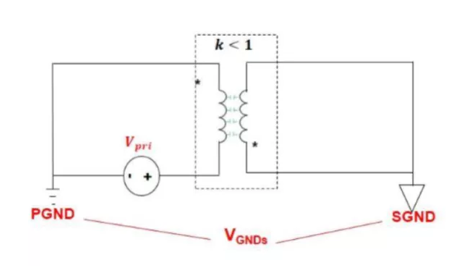Figure 7: Primary and Secondary Model of VPRI as a Noise Source

A network analysis model can be used to obtain the various parameters in the impedance network. Figure 8 shows the measurement method of the transformer’s CM impedance, where the network analysis model applies excitation on both ends of the primary-side switch. The port’s S-parameters between the primary-side and secondary-side ground can be measured.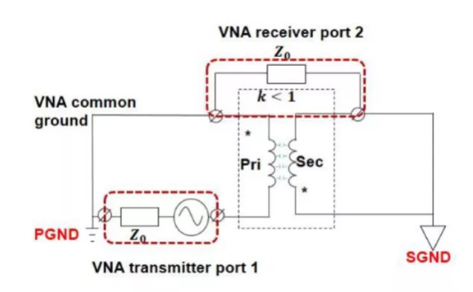Figure 8: Transformer’s CM Impedance Measurement Method

Figure 9 shows the π model. This model represents the impedance network between the ports, based on the measured scattering parameters.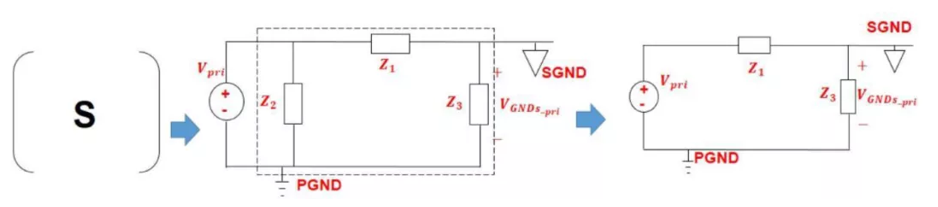Figure 9: Transformer’s High-Frequency Impedance Model

In this network, Z2 can be ignored because the impedance in parallel with the voltage source is negligible. The equivalent CM voltage and CM impedance produced by VPRI can be estimated. VCMPRI can be calculated using Equation (1):

$$V_{CMPri} = V_{Pri} \times CMTG_{Pri} = V_{Pri} \times\frac{Z_3}{Z_1+Z_3}$$

Where CMTGPRI is the primary voltage source’s transfer function in relation to the CM noise source. ZCMTRANSPRI can be calculated using Equation (2):

$$Z_{CMTransPri} = \frac{Z_3}{Z_1+Z_3}$$

Simply reducing the transformer’s impedance is not necessarily the most effective approach to reduce radiation. The best method is to minimize the Z3 to Z1 ratio using a balanced transformer design. Part III will cover this design criteria to ensure accurate measurement results.

## Conclusion

This article introduced a ferrite bead solution to avoid CM current measurement errors that arise from the influence of coupling and grounded impedance. In continuation of our analysis of measuring high-frequency, CM current, we also covered how to obtain CM impedance using a network analysis model, π model, and by calculating the primary CM voltage.

In Part III, we will discuss the switching noise effect and equivalent voltage source, then confirm our proposed measurement approaches.